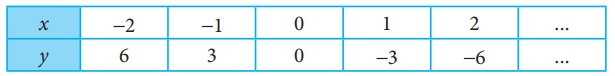Home | | Maths 7th Std | Exercise 5.1 (Tables and Patterns Leading to Linear Functions)

# Exercise 5.1 (Tables and Patterns Leading to Linear Functions)

7th Maths : Term 2 Unit 5 : Information Processing : Text Book Back Exercises Questions with Answers, Solution

Exercise 5.1

1. Match the given patterns of shapes with the appropriate number pattern and its generalization.i) (d)

ii) (a)

iii) (e)

iv) (c)

v) (b)

Objective type questions

2. Identify the correct relationship between x and y from the given table.(i) y = 4x

(ii) y = x + 4

(iii) y = 4

(iv) y = 4 ├Ś 4

3. Identify the correct relationship between x and y from the given table.(i) y = ŌłÆ2x

(ii) y = +2x

(iii) y = +3x

(iv) y = ŌłÆ3x

Exercise 5.1

1. (i) (d)

(ii) (a)

(iii) (e)

(iv) (c)

(v) (b)

Objective type questions

2.(i) y = 4x

3. (iv) y = ŌłÆ3x

Tags : Information Processing | Term 2 Chapter 5 | 7th Maths , 7th Maths : Term 2 Unit 5 : Information Processing
Study Material, Lecturing Notes, Assignment, Reference, Wiki description explanation, brief detail
7th Maths : Term 2 Unit 5 : Information Processing : Exercise 5.1 (Tables and Patterns Leading to Linear Functions) | Information Processing | Term 2 Chapter 5 | 7th Maths